# A fraction

I think of a fraction, if we increase its numerator and denominator by one, the value of the fraction increases by one tenth, what fraction do I think?

x =  0.4

### Step-by-step explanation:

Our quadratic equation calculator calculates it.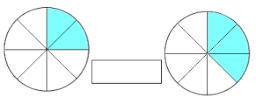Did you find an error or inaccuracy? Feel free to write us. Thank you!Tips to related online calculators
Looking for help with calculating roots of a quadratic equation?
Need help to calculate sum, simplify or multiply fractions? Try our fraction calculator.
Do you solve Diofant problems and looking for a calculator of Diofant integer equations?
Do you have a linear equation or system of equations and looking for its solution? Or do you have a quadratic equation?

## Related math problems and questions:

• Over fractionSolve equation: p over 5 equals fraction numerator 7 p over denominator 6 end fraction plus 5
• Guess a fractionTom was asked to guess a fraction. The sum of 1/2 the numerator and 1/3 of its denominator is 30. If Tom subtracts 36 from its denominator, the fraction becomes 1/3. What is the fraction that Tom was asked to guess? (Leave your answer in simplest form)
• When 5When 5 is subtracted from both my numerator and denominator I become 3/4. What fraction i am?
• Unknown number 4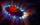I think number. When I divided its square root by its 1/9, I get a number 1. What number am I thinking?
• Division by zero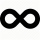Fraction 5 by 2. if 3 is added to numerator and 2 is subtracted from the denominator then the new fraction is:
• Volume of sphereHow many times does the volume of a sphere increase if its radius increases 2 ×?
• Denominator 2Denominator of a fraction is 5 and numerator is 7. Write the fraction .
• Square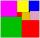If we increase one side of square by its one-half then square perimeter increase by 10 cm. What is the side of the square?The product of the two numbers is 900. If we increase the lowest number by two, then its product will increase by 150. Determine both numbers.
• Find theFind the number x, which if it increases by 2, then its square increases by 21 percent.
• Square Number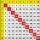If to a square of integer number add 17, we get the square of the next integer number. What is the original number?
• Value of expressionX=2, y=-5 and z=3 what is the value of x-2y?
• Fraction unknowns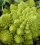Divide of fractions with unknowns: Fraction 1: The quantity x squared plus 6 times x plus 9 over the quantity x minus 1. Fraction 2: the quantity x squared minus 9 over the quantity x squared minus 2 times x plus 1.  Find Fraction 1 over Fraction 2.
• Cylinder twiceIf the radius of the cylinder increases twice, and the height is reduced twice, then the volume of the cylinder increases (how many times?):
• Two fraction equation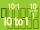Find d value of q in the equation 30/16=q/48.How many times does the surface of a sphere decrease if we reduce its radius twice?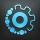A 20 percent price increase meant a 90-crown raise. How many cost a product after?